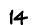...the official site for results from the National Assessment of Educational ProgressHome## Mathematics Sample QuestionsThree sample mathematics questions are presented in this section for each age. The response options for multiple-choice questions are provided as the students saw them, and the oval for the correct answer is filled in. All constructed-response questions in the long-term trend mathematics assessment were scored as correct or
incorrect, and the correct response is shown on the answer line.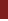Age 9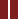Age 13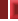Age 17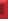Sample Question 1Sample Question 2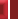Sample Question 3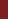Sample question 2 asked students to demonstrate procedural knowledge by evaluating a function for a given value.

 65 percent of 17-year-olds answered this question correctly.

If f (z) = z + 8, what is the value of f (6)?

 The correct answer is: